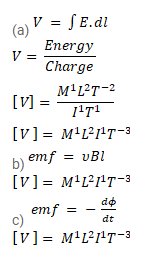# Calculate the dimensions of

Question:

Calculate the dimensions of

(a) $\int \vec{E} \cdot \overrightarrow{d l}$

(b) $v B l$ and

(c) $\frac{d \varphi_{S}}{d t}$

The symbols have their usual meanings

Solution: